Search for content and authors

## Method for determining chemical vapor deposition occurrence using langasite crystal microbalance

Hitoshi Habuka ,  Misako Matsui ,  Ayumi Saito

Yokohama National University (YNU), 79-5 Tokiwadai Hodogaya, Yokohama 240-8501, Japan

 Abstract In order to develop the method determining the existence of surface chemical reactions, the frequency behaviour of the langasite crystal microbalance (LCM) set in a chemical vapour deposition (CVD) reactor was studied, in detail. The parameter, C(T), of the equation for expressing the LCM frequency change, (: gas density, : gas viscosity), was evaluated. In a hydrogen-monomethylsilane system, the LCM frequency very gradually increased after the monomethylsilane gas introduction above 500 oC, although that at lower temperatures was maintained flat. The difference of C(T) values in monomethylsilane-hydrogen system from  those in nitrogen-hydrogen system increased above 500 oC. Thus, the silicon carbide film deposition from monomethylsilane gas was determined to begin near 500 oC. 1. Introduction Chemical vapour deposition (CVD) is the key technology  for producing various useful thin and thick films. When a new film deposition process is designed and developed, particularly employing new combinations between precursors, reactors and process conditions, the information on the occurrence of surface chemical reactions is often useful by some reasons. For surveying the parameters producing significantly thin films, nanometre-thick or monolayer-thick, the critical temperature to initiate the surface chemical reaction can be key information. The success of the thin film formation can be validated typically by the transmission electron microscope (TEM), deposition by deposition . However, because this technique is often expensive, an alternative low cost and easy method should be developed. The temperature to initiate the surface chemical reaction is useful information, for designing and maintaining the practical thermal condition of the cold wall reactor. In a cold wall system, the substrate is very often heated using the infrared lamps from the outside of the transparent quartz vessel. When the film deposition occurs at the reactor wall, the formed film significantly absorbs the infrared light to increase the quartz wall temperature; the cold wall thermal environment cannot be maintained. Additionally, the film formed at the reactor inner wall often emits small particles to cause hillocks and various defects on the CVD film surface. Thus, in order to avoid these troubles, the reactor wall temperature must be maintained below the acceptable highest temperature, that is, the critical temperature, at which the surface chemical reactions by the precursors are completely suppressed. The expected solution for detecting the surface chemical reaction occurrence is the in-situ monitoring technique using the langasite crystal microbalance (LCM) [2-4], because the LCM has a potential for sensitively working at low and high temperatures in various inactive and reactive gas ambient atmosphere. As shown in our previous studies [2, 4], the LCM has the potential to detect the various small changes in the heat and flow conditions in a reactor, caused by the nanometre-thick film deposition. In our extended study , parameters of the equation [6, 7] were optimized so that the LCM frequency behaviour could be simply expressed using the gas properties in an ambient of gas mixture of hydrogen and nitrogen. Next to be performed is the development of the systematic method which can detect significantly small change caused by the surface chemical reaction from the LCM behaviour in very wide ranges of the CVD conditions. In this study for determining the surface chemical reaction occurrence, the LCM frequency behaviour was evaluated using gases, such as nitrogen and monomethylsilane in ambient hydrogen, over wide ranges of temperature and gas concentrations. The difference of the LCM frequency behaviours between the ambient containing nitrogen gas and monomethylsilane gas and that between the high and low temperatures was studied in detail. 2. Experimental procedure and equation The horizontal cold wall reactor containing the LCM, shown in Fig. 1, was used. This reactor consists of a gas supply system, a quartz chamber and infrared lamps. The gas supply system can introduce hydrogen, nitrogen and monomethylsilane gases. Hydrogen is the carrier gas. The gas flow channel of this reactor has a low height and a small rectangular cross section in order to achieve a high consumption efficiency of the reactive gases. The height and the width of the quartz chamber were 10 mm and 40 mm, respectively, similar to those used in our previous studies [2, 4, 5, 8-12]. A 30-mm-wide x 40-mm-long (100) silicon wafer manufactured by the Czochralski method was horizontally placed on the bottom wall of the quartz chamber. The LCM (Halloran Electronics, Tokyo, Japan) was placed 5 mm above the silicon wafer and was connected to a personal computer in order to record its frequency. The LCM has an intrinsic frequency of 10 MHz similar to our previous studies [2, 4, 5]. The silicon wafer and the LCM were simultaneously heated by infrared light from halogen lamps through the quartz chamber. The LCM was heated not only by the infrared light, but also by radiation heat and conduction heat from the hot silicon wafer. The temperature of the silicon wafer was measured prior to the CVD process using thermocouples. Because the position of the LCM was very near the silicon wafer, the temperature of the LCM was assumed to be the same as that of the silicon wafer.  A typical process used in this study is shown in Fig. 2. First, the LCM was heated to 300 - 600 oC in ambient hydrogen at atmospheric pressure. After waiting until the LCM frequency became stable, the nitrogen gas and monomethylsilane gas were introduced at atmospheric pressure into the reactor chamber. The flow rates of the nitrogen gas and monomethylsilane gas were 0 – 1.0 slm and 0.05 - 0.3 slm, respectively. The total gas flow rate was adjusted to 1 slm. In the previous studies [5, 6], the parameters of equation for expressing the LCM frequency change using the fluid properties were optimized to linearly express the LCM frequency difference of the gas mixture from the ambient hydrogen, as Δfgas=-C(T)ρ Mixy ηMixy.                                                            (1) C(T)=A e(-B/T).                                                                           (2) where the y and z values were 1.3 and 1.3, respectively. The C(T) value was expressed in a form of Arrhenius type equation, as Eq. (2), when any chemical reaction did not occur. Any chemical reactions for the film formation are expected to sensitively influence the C(T) value. 3. Results and discussion 3.1 LCM frequency First, the LCM frequency behaviours at various conditions were measured and evaluated in the monomethylsilane-hydrogen system. In order to recognize the entire behaviour of the LCM frequency, the LCM frequency differences from hydrogen ambient at 300 - 600 oC was shown in Fig. 3. Immediately after opening the gas valve at 0 s for the monomethylsilane gas supply, the monomethylsilane gas at high concentration was introduced into the reactor, because the monomethylsilane gas at 100 % remained between the gas valve and the mass flow controller came to the reactor. During the high concentration gas passing through the reactor, the LCM frequency significantly decreased. After this, the monomethylsilane gas, which concentration was adjusted to the set value, reached the LCM in the reactor. Thus, the fluctuation of the LCM frequency became small. As shown in Fig. 3, the LCM frequency difference measured at 300 oC was very stable after 20 s. This indicated that the gas temperature and the fluid properties, such as gas density and gas viscosity, were in a steady state. This simultaneously indicated that there was no chemical reaction at 300 oC, because of no thermal change caused by no reaction heat. The behaviour at 400 and 500 oC was similar to that at 300 oC. The trend of LCM frequency difference at 300, 400 and 500 oC was the same and parallel to each other. Thus, the monomethylsilane-hydrogen system below 500 oC is concluded to have no chemical reaction. Although the LCM frequency difference at 550 oC seemed to be relatively stable, it very gradually increased after 20 s. Similar to this, the LCM frequency difference at 600 oC also slightly increased with the larger gradient than that at 550 oC. This gradual increase in the LCM frequency difference indicated that any transient change relating to chemical reaction, such as temperature, gas density and gas viscosity, occurred and continued in the reactor during the introduction of monomethylsilane gas. As reported in our previous study  and as shown in Fig. 3, the LCM frequency increased with the decreasing temperature. Additionally, the surface chemical reaction for silicon carbide film deposition from monomethylsilane gas is endothermic . The frequency decrease due to the weight increase by the film deposition was overcompensated by the frequency increase due to the temperature decrease by the endothermic reaction. Thus, the LCM frequency could continue to increase till reaching the steady state. From Fig. 3, the chemical reaction occurring at the LCM surface is considered to continue after 200 s. The temperature change should be enhanced by the increasing reaction rate, because of the greater reaction heat. In order to clearly show this trend, the LCM frequency gradient at various temperatures in the monomethylsilane-hydrogen system was evaluated, as shown in Fig. 4. As shown in this figure, the LCM frequency gradient in the low temperature range between 300 – 500 oC was less than zero Hz/s. This value should be recognized to show the state with no chemical reaction. In contrast, the LCM frequency gradient increased at 550 oC from the value less than zero to the positive value of 2 Hz/s. It further increased to the value higher than 4 Hz/s at 600 oC. Because the LCM frequency gradient increased with the increasing temperature, the surface chemical reaction at the LCM surface was initiated in the temperature range between 500 and 600 oC. 3.2 C(T) Parameter In the previous section, the chemical reaction occurrence could be determined using the LCM frequency difference from that measured in the ambient having no reactive gases. However, the LCM frequency is significantly sensitive to the fluctuation of various parameters. Thus, in this section, a systematic method to find the occurrence of the surface chemical reaction is studied. Because the chemical reaction induces various changes, such as the temperature, gas composition and fluid properties influencing the LCM frequency, the relationship of Eq. (1) between the LCM frequency and the gas properties is evaluated. Fig. 5 shows the LCM frequency change with the increase in  of the gas mixture of monomethylsilane, nitrogen and hydrogen at 400, 500 and 600 oC. In this figure, the frequency differences of nitrogen-hydrogen system (white circles) follows the Eq. (1), shown as a linear relationship. The monomethylsilane-hydrogen system (triangles) at 400 and 500 oC coincided with the behavior of nitrogen-hydrogen system. In contrast to this, the monomethylsilane-hydrogen system showed a slightly large gradient in the region of small value. Thus, the C(T) value of Eq. (1) is expected to indicate the surface chemical reaction occurrence. The C(T) values obtained using Eq. (1) are plotted against 1/T. as shown in Fig. 6. This figure shows that the C(T) values for nitrogen-hydrogen system entirely have a linear relationship following Eq. (2), at the temperatures from 300 to 600 oC. In contrast, the monomethylsilane-hydrogen system shows the increase of the C(T) value at the higher temperatures. In order to clearly understand the behavior difference of the C(T) values between monomethylsilane and nitrogen, the C(T) value difference of monomethylsilane-hydrogen system from nitrogen-hydrogen system are shown in Fig. 7. As shown in this figure, the C(T) value difference at the temperatures lower than 500 oC were around zero. This concludes the monomethylsilane gas at less than 500 oC was the same as that of nitrogen which had no chemical reaction. The monomethylsilane-hydrogen system showed the C(T) value increase to higher than 1 x 1010 at the temperatures between 500 and 550 oC. Because these values were obtained being separated from the fluid properties, the C(T) value change could be understood as the additional phenomena occurring at the higher temperatures, such as the surface chemical reaction for the film deposition. As reported in our previous study  using the LCM and TEM, the nanometre-thick silicon carbide film could be formed at 600 oC from monomethylsilane gas. Taking into account that the film deposition rate significantly decreases with the 100 K decrease of the substrate temperature , the silicon carbide film produced around 500 oC is expected to be less than nanometre- or angstrom-thick. Thus, the temperatures near 500 oC can be the critical temperature for the silicon carbide film deposition from monomethylsilane gas. 4. Conclusions In order to develop the method to determining the occurrence of the surface chemical reaction for the chemical vapour deposition, the LCM frequency behaviour was studied in detail. The LCM frequency immediately after the introduction of the monomethylsilane gas gradually increased at the temperature higher than 500 oC. The gradient of the LCM frequency increased with the temperature higher than this temperature. Simultaneously, the parameter, C(T), to describe the LCM frequency difference in the monomethylsilane-hydrogen system consistently showed a discontinuity between the temperatures above and below 500 oC. Additionally, the prediction of nanometre- or angstrom-thick film deposition by the TEM, previously obtained, was consistent with this result. Thus, the method developed in this study is expected to determine the critical temperature of the surface chemical reaction for the chemical vapour deposition. Acknowledgement The authors would like to thank Mr. Nobuyoshi Enomoto of Halloran Electronics Co., Ltd., for his technical support.    References  S. M. Sze, Semiconductor devices: physics and technology. (2008) John-Wiley, Hoboken, NJ, USA.  H. Habuka and Y. Tanaka, J. Surf. Eng. Mater. Adv. Tech., 3 (2013) 61-66.  M. Schulz, J. Sauerwald, H. Seh H. Fritze, H. L. Tuller, Solid State Ionics 184 (2011) 78-82.  H. Habuka and Y. Tanaka, ECS J. Solid State Sci. Tech., 1 (2012), 62-65.  H. Habuka and M. Matsui, EuroCVD19, (Varna, Bulgaria, 2013), accepted.  K. K. Kanazawa and J. G. Gordon II, Anal. Chim. Acta, 175 (1985) 99-105  G. Sauerbrey, Zeitschrift fur Physik, 155 (1959) 206-222.  H. Habuka and K. Kote, Jpn. J. Appl. Phys. 50 (2011) 096505-1-4.  H. Habuka H. Ohmori and Y. Ando, Surf. Coat. Tech., 204 (2010) 1432-1437.  H. Habuka, and Y. Ando, J. Electrochem. Soc., 158 (2011) H352-H357.  H. Habuka, M. Watanabe, Y. Miura, M. Nishida, T. Sekiguchi, J. Crystal Growth, 300 (2007) 374-381.  H. Habuka, M. Watanabe, M. Nishida and T. Sekiguchi, Surf. Coat. Tech., 201 (2007) 8961-8965.  H. Habuka, T. Nagoya, M. Mayusumi, M. Katayama, M. Shimada and K. Okuyama, J. Crystal Growth, 169 (1996) 61-72.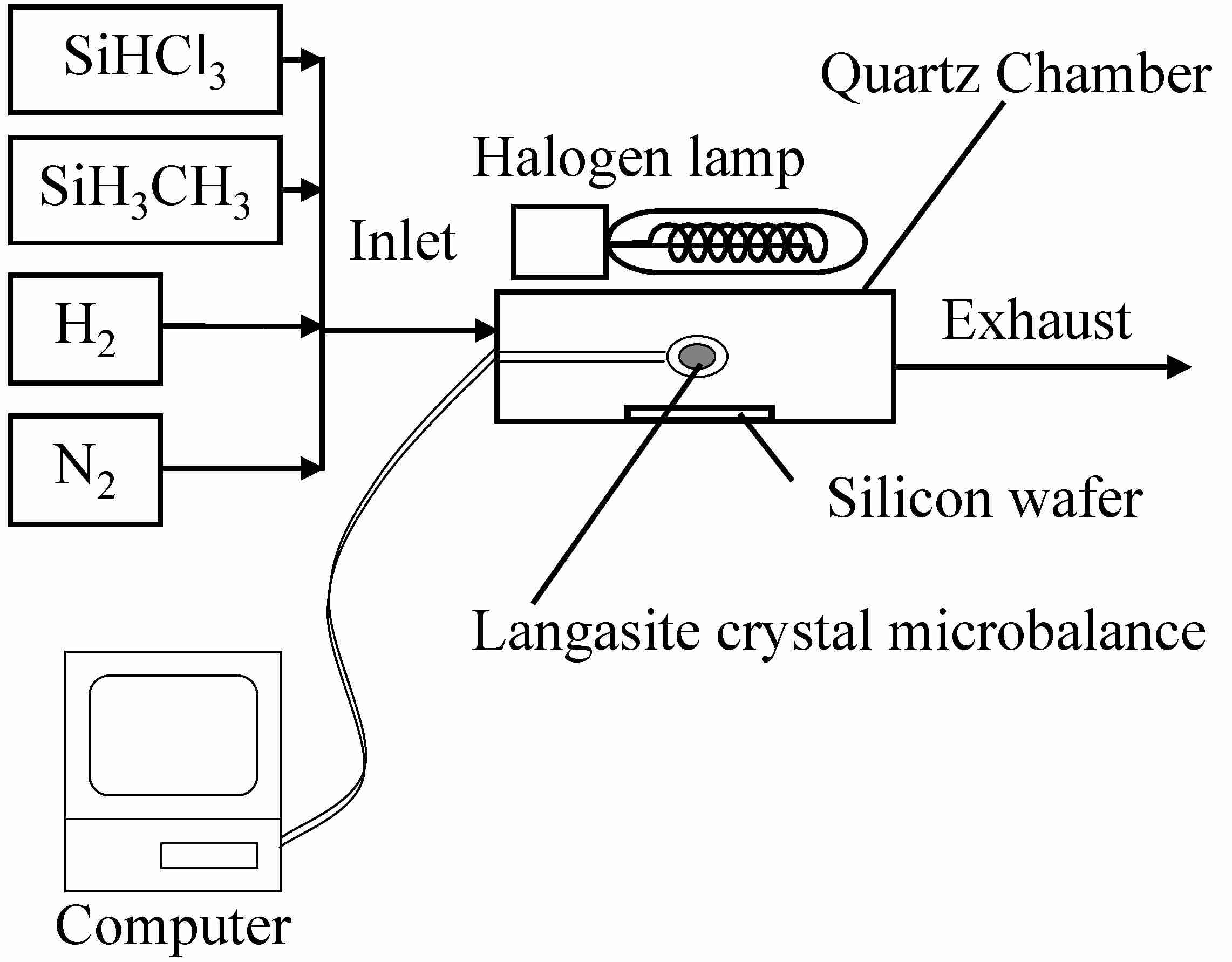Figure 1 Cold wall chemical vapor deposition reactor containing the LCM system.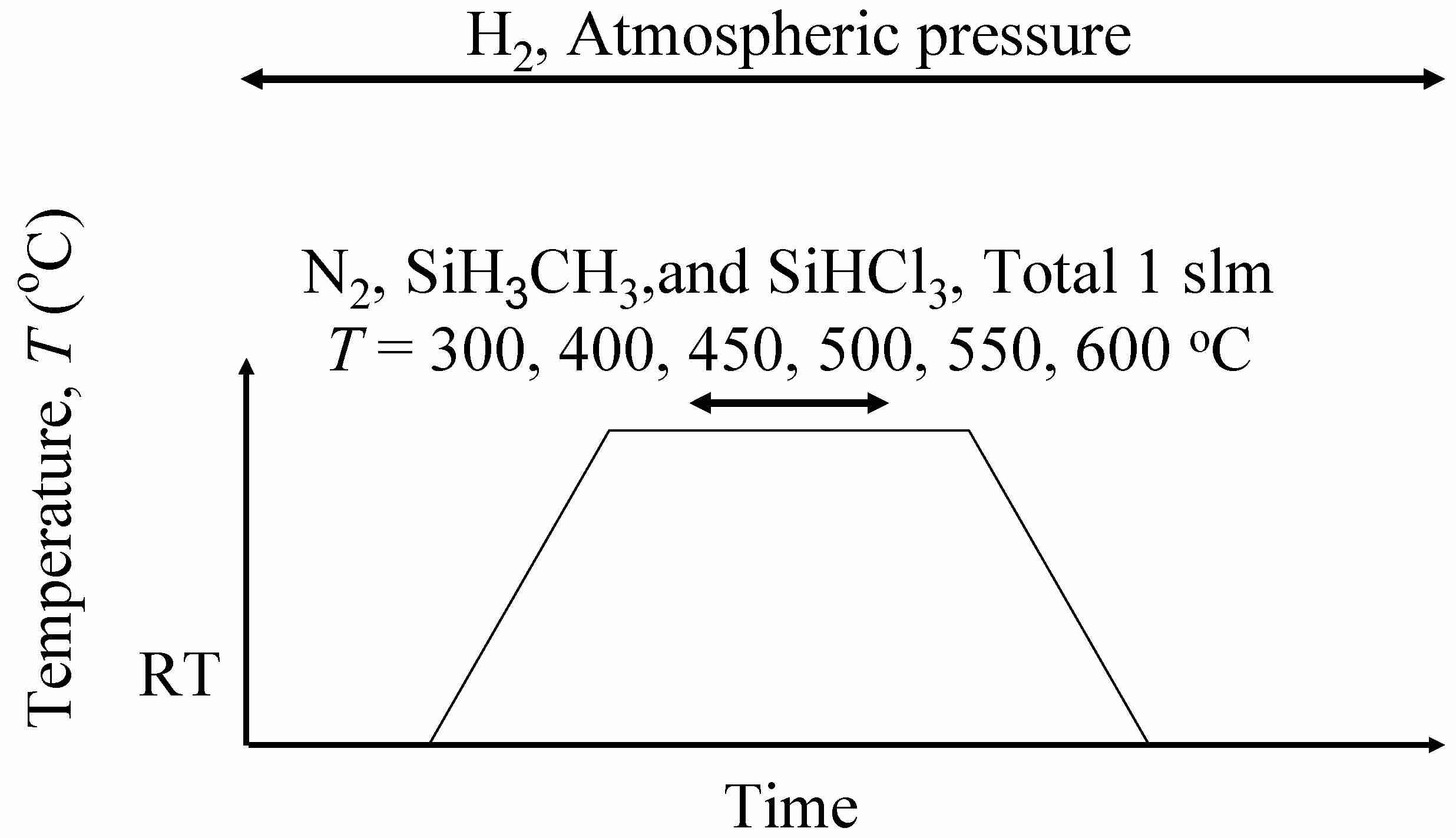Figure 2 Process for measuring the LCM frequency using various gases.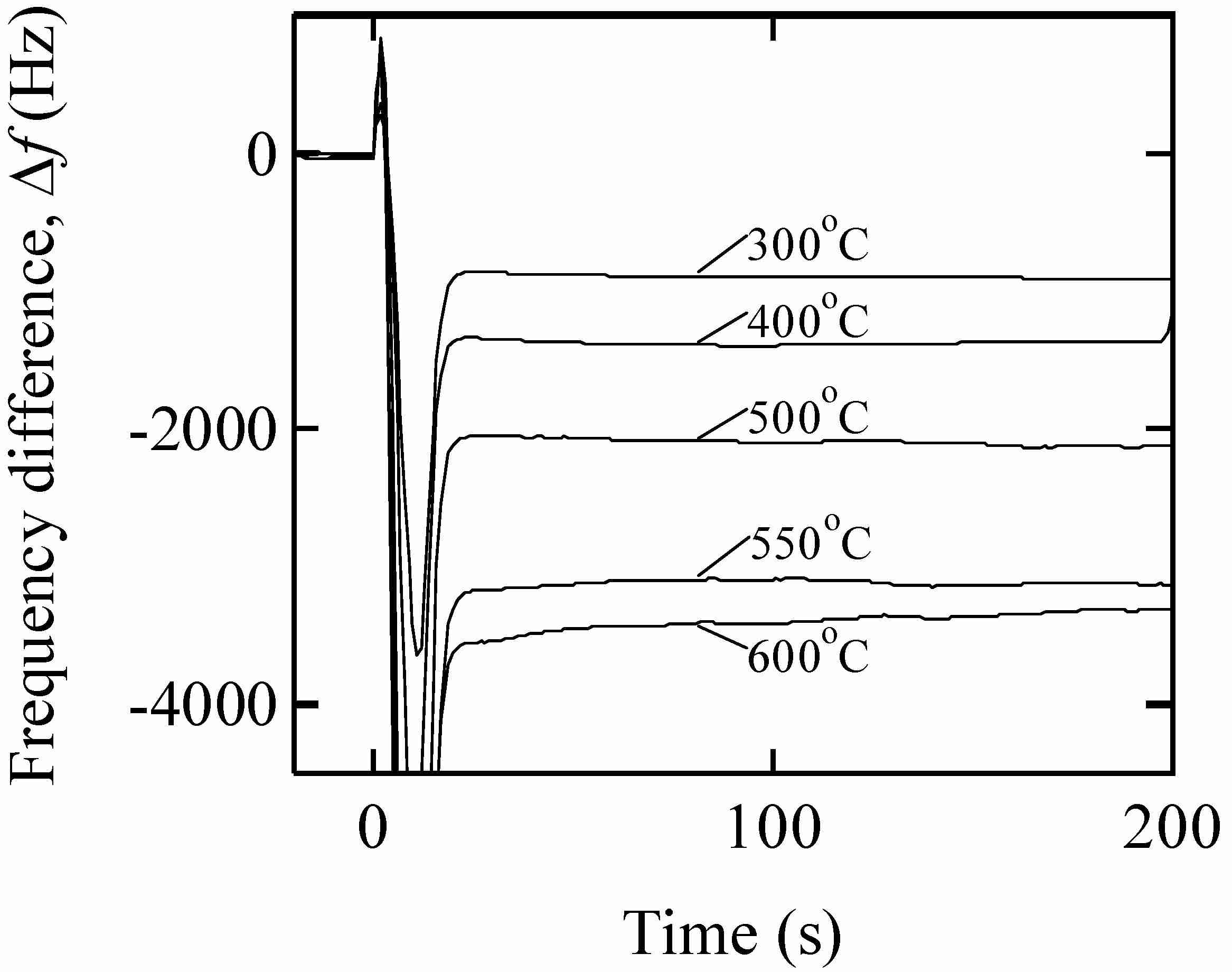Figure 3　The LCM frequency change immediately after the introduction of monomethylsilane gas to hydrogen ambient atmosphere.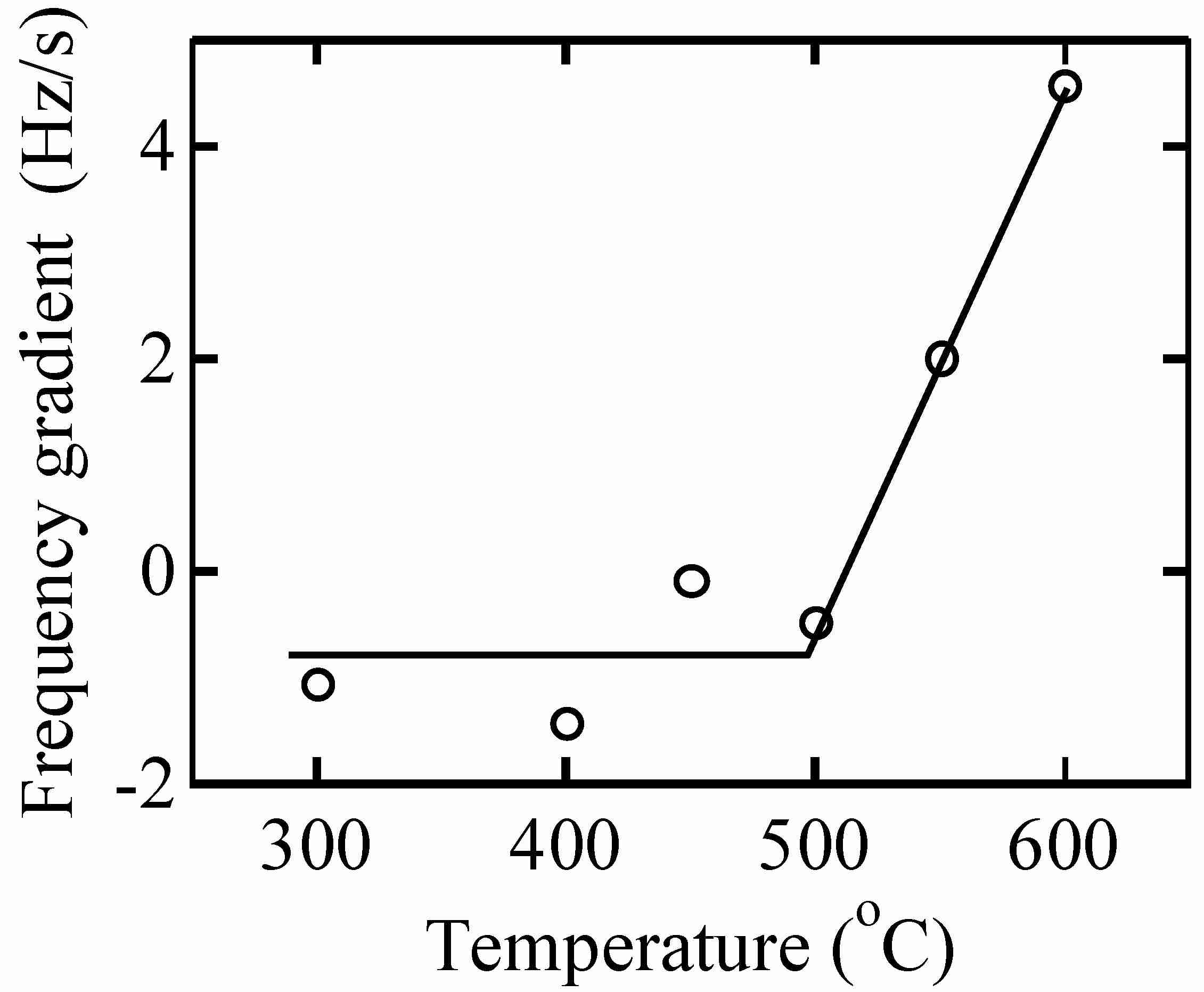Figure 4 LCM frequency gradient immediately after introducing the monomethylsilane gas at various temperatures.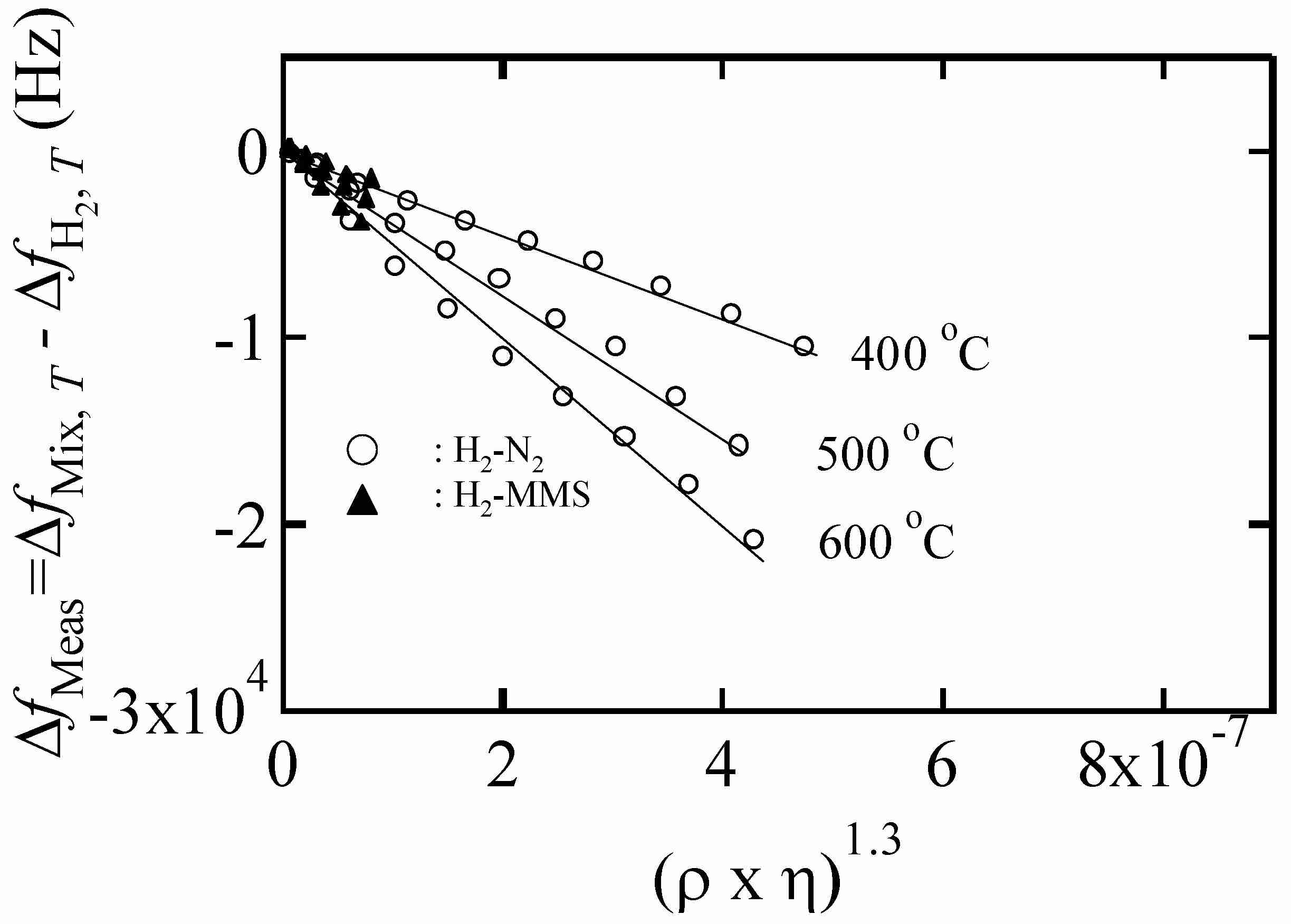Figure 5 LCM frequency difference of monomethylsilane gas (dark triangle) and nitrogen gas (circle) from hydrogen ambient.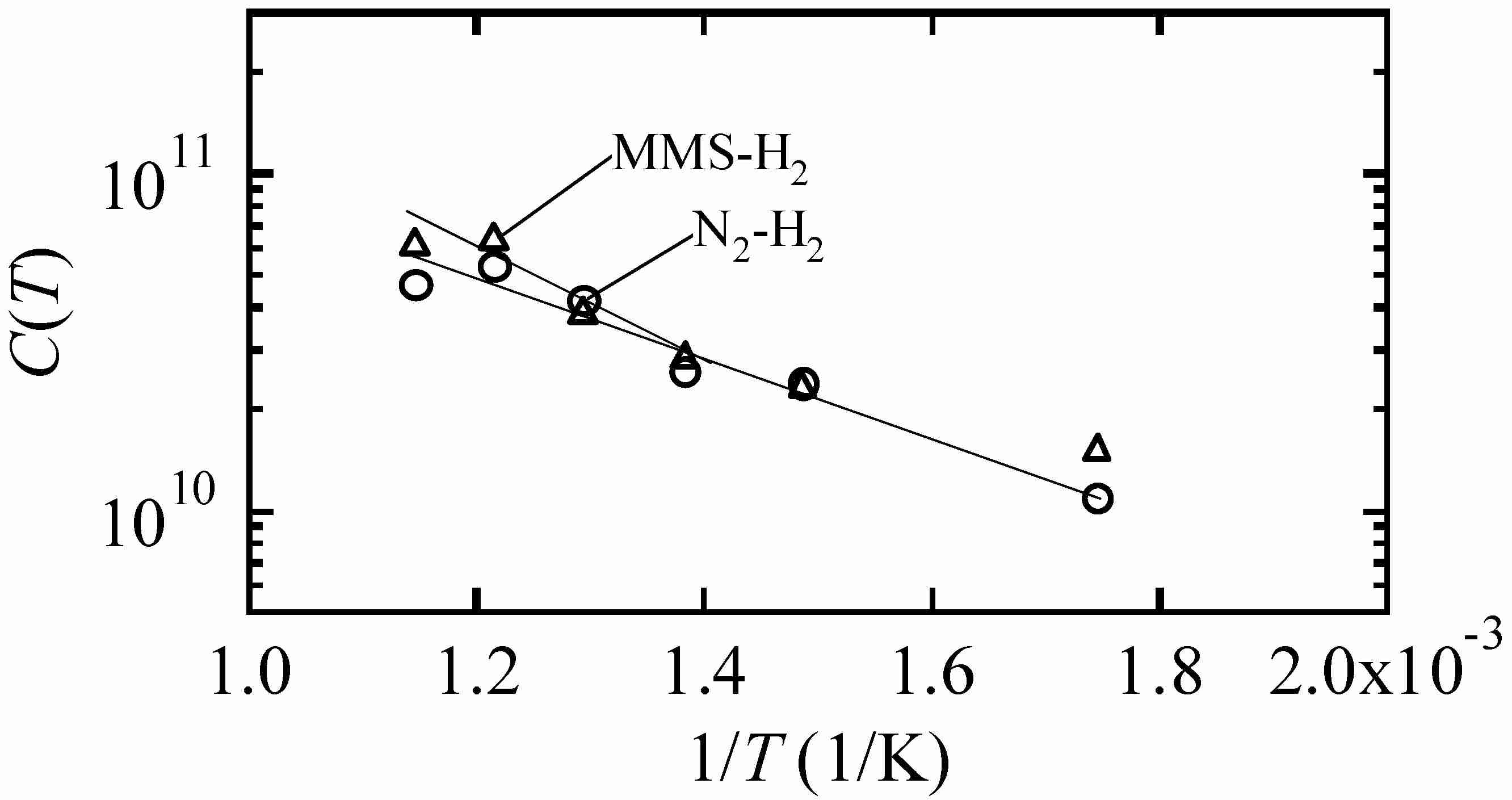Figure 6 C(T) values of monomethylsilane-hydrogen system (triangles) and nitrogen-hydrogen system (circles).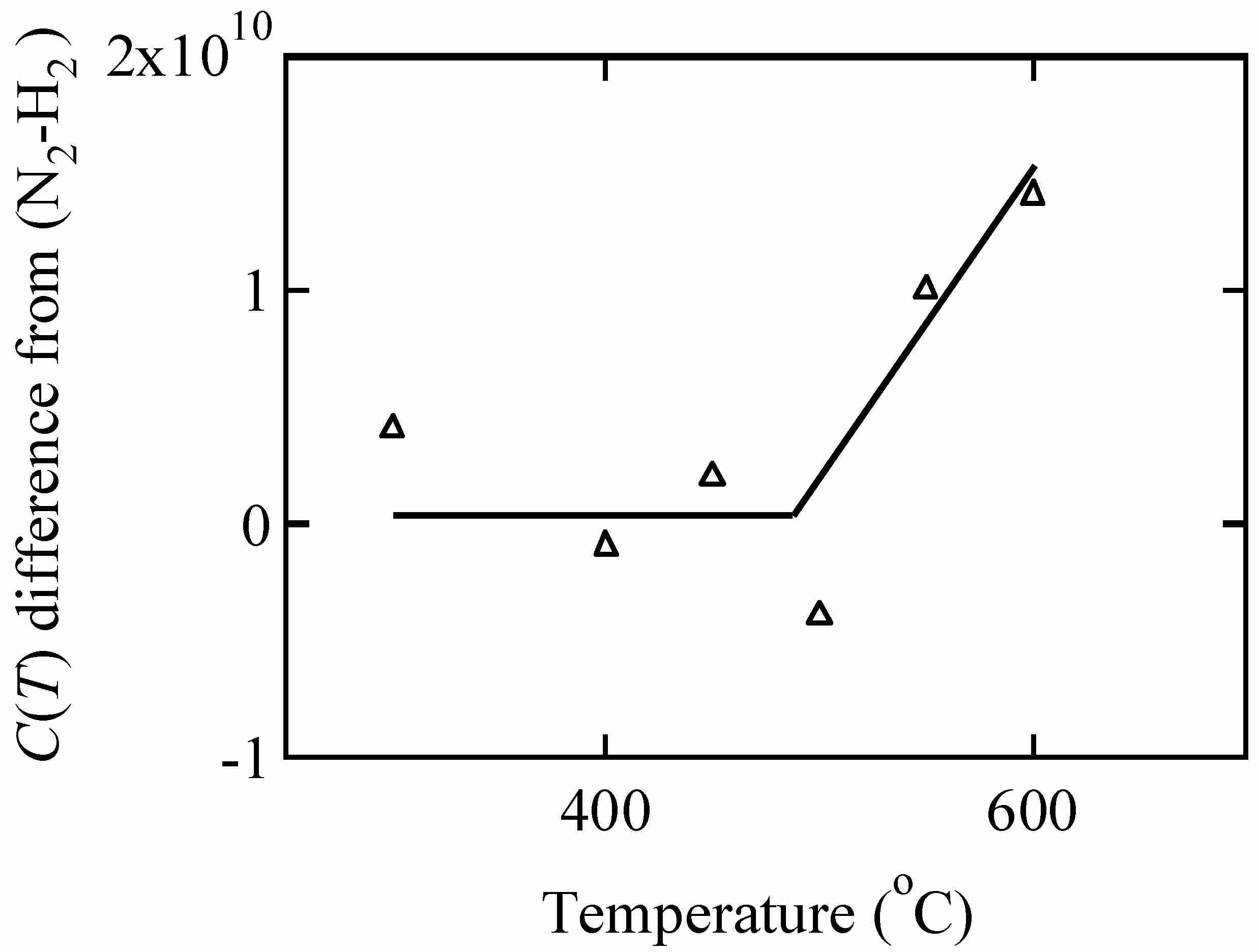Figure 7 C(T) value difference of monomethylsilane-hydrogen system from nitrogen-hydrogen system.

Legal notice
• Legal notice:

Related papers